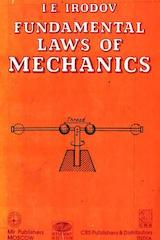# Fundamental Laws of Mechanics by I.E. IrodovApart from Problems in General Physics, IE Irodov also published two related books (1) Fundamental Laws of Mechanics and (2) Basic Laws of Electromagnetism. This article reviews Fundamentals of Mechanics by IE Irodov.

The book consists of two parts (I) five chapters of classical mechanics and (II) two chapters of relativistic mechanics. This review is limited to the book's utility for IIT JEE. Hence, we focus on the initial five chapters, which are

1. Essentials of Kinematics
2. The Basic Equation of Dynamics
3. Energy Conservation Law
4. The Law of Conservation of Momentum
5. The Law of Conservation of Angular Momentum
Each chapter begins with a concise theory followed by a number of the instructive and interesting problems.

## Utility of this Book for IIT JEE

The book contains standard problems, all solved, and most of them are in JEE syllabus (except a few involving rotating frames). JEE aspirants are advised to go through these problems (approximately 80 of them). Techniques like solutions to collision problems in Centre of Mass frame and Vector diagram of momentum are very useful. Most of these problems are also included in Irodov's well-known book Problems in General Physics.

The theory part is concise and to the point (terse), as in most of Russian physics books. The use of vectors and vector calculus further complicates it. It excludes all things of minor importance and concentrate on the concepts/questions which are the hardest. Also, a few sections are out of JEE syllabus. My advice is to skip it or read it selectively.